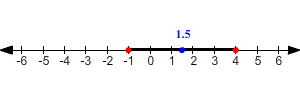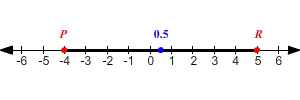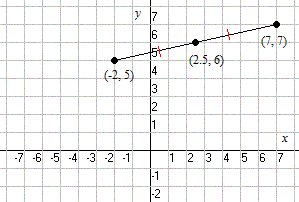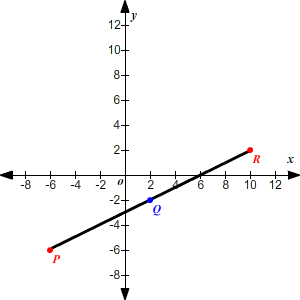# The Midpoint Formula

## In One Dimension

On a number line , the number halfway between ${x}_{1}$ and ${x}_{2}$ is

$\frac{{x}_{1}+{x}_{2}}{2}$

Example 1:

Find the midpoint between $-1$ and $4$ .

Use the formula. The midpoint is

$\frac{-1+4}{2}=\frac{3}{2}=1.5$ .Example 2:

If $0.5$ is the midpoint of $\stackrel{¯}{PR}$ and the coordinate of $P$ is $-4$ , find the coordinate of $R$ .

Use the formula.

$\frac{-4+{x}_{2}}{2}=0.5$

To begin solving, multiply both sides by $2$ .

$-4+{x}_{2}=1$

Next, add $4$ to both sides.

${x}_{2}=5$

So, the coordinate of $R$ is $5$ .## In Two Dimensions

Suppose you are given two points in the plane ( ${x}_{1}$ , ${y}_{1}$ ) and ( ${x}_{2}$ , ${y}_{2}$ ), and asked to find the point halfway between them. The coordinates of this midpoint will be:

$\left(\frac{{x}_{1}+{x}_{2}}{2},\frac{{y}_{1}+{y}_{2}}{2}\right)$

An easy way to think about this is that the $x$ -coordinate of the midpoint is the average of the $x$ -coordinates of the two points, and likewise with the $y$ -coordinate.

Example 1:

Find the midpoint between $\left(-2,5\right)$ and $\left(7,7\right)$ .

Use the formula. The coordinates of the midpoint are:

$\left(\frac{-2+7}{2},\frac{5+7}{2}\right)$

Simplify.

$\left(2.5,6\right)$Example 2:

If $Q\left(2,-2\right)$ is the midpoint of $\stackrel{¯}{PR}$ and $P$ has the coordinates $\left(-6,-6\right)$ , find the coordinates of $R$ .

Use the formula to write and solve two equations for the coordinates of $R$ .

$Q\left(2,-2\right)=\left(\frac{-6+{x}_{2}}{2},\frac{-6+{y}_{2}}{2}\right)$

First, find the $x$ -coordinate.

$\begin{array}{l}2=\frac{-6+{x}_{2}}{2}\\ 4=-6+{x}_{2}\\ 10={x}_{2}\end{array}$

Then, find the $y$ -coordinate.

$\begin{array}{l}-2=\frac{-6+{y}_{2}}{2}\\ -4=-6+{y}_{2}\\ \text{\hspace{0.17em}}\text{\hspace{0.17em}}\text{\hspace{0.17em}}2={y}_{2}\end{array}$

So, the coordinates of $R$ are $\left(10,2\right)$ .## In Three Dimensions

It's pretty easy to guess based on the formula for two dimensions!

In $3$ -dimensional space, the midpoint between ( ${x}_{1}$ , ${y}_{1}$ , ${z}_{1}$ ) and ( ${x}_{2}$ , ${y}_{2}$ , ${z}_{1}$ ) is

$\left(\frac{{x}_{1}+{x}_{2}}{2},\frac{{y}_{1}+{y}_{2}}{2},\frac{{z}_{1}+{z}_{2}}{2}\right)$